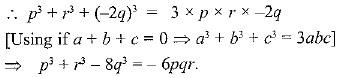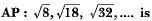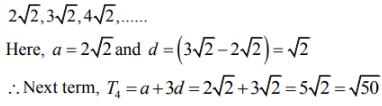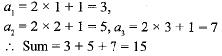Courses

# Important Questions: Arithmetic Progressions

## 10 Questions MCQ Test Mathematics (Maths) Class 10 | Important Questions: Arithmetic Progressions

Description
This mock test of Important Questions: Arithmetic Progressions for Class 10 helps you for every Class 10 entrance exam. This contains 10 Multiple Choice Questions for Class 10 Important Questions: Arithmetic Progressions (mcq) to study with solutions a complete question bank. The solved questions answers in this Important Questions: Arithmetic Progressions quiz give you a good mix of easy questions and tough questions. Class 10 students definitely take this Important Questions: Arithmetic Progressions exercise for a better result in the exam. You can find other Important Questions: Arithmetic Progressions extra questions, long questions & short questions for Class 10 on EduRev as well by searching above.
QUESTION: 1

Solution:
QUESTION: 2

### If p, q, r are in AP, then p3 + r3 - 8q3 is equal to

Solution:

∵ p, q, r are in AP.
∴ 2 q = p + r
⇒ p + r - 2 q = 0QUESTION: 3

### The next term of theSolution:

The given AP is √8, √18, √32,......... On simplifying the terms, we get:QUESTION: 4

In an AP, if a = 3.5, d = 0, n = 101, then an will be

Solution:

a101 = 3.5 + 0 (100) = 3.5

QUESTION: 5

The famous mathematician associated with finding the sum of the first 100 natural numbers is

Solution:

Johann Friedrich Gauss, he was a German mathematician who find the sum of the first 100 natural number.

QUESTION: 6

The list of numbers -10, -6, -2, 2, ... is

Solution:
QUESTION: 7

The 6th term from the end of the AP: 5, 2, -1 , -4 , . . . , -31 is

Solution:
QUESTION: 8

Two APs have the same common difference. The first term of one of these is -1 and that of the other is -8. Then the difference between their 4th terms is

Solution:

a4 - b4 = (a1 + 3d) - (b1 + 3d)
= a1 b1 = - 1 - (-8) = 7

QUESTION: 9

Which term of the AP : 21, 42, 63, 84, ... is 210 ?

Solution:
QUESTION: 10

If the nth term of an AP is (2n + 1), then the sum of its first three terms is

Solution: